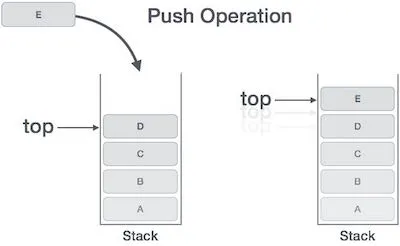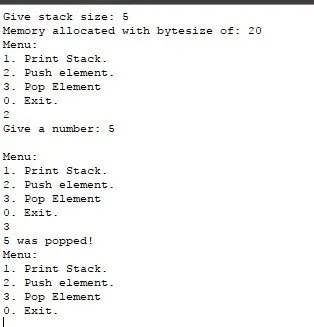# Programming - Assembly Stack DatastructureHello it's me again! Today, after a long time of not uploading Assembly, even though I said that some Examples will come from time to time, I present you with a Stack Implementation using a Dynamic Array that gets set in the start of the programm! Just to point it out this Code is not perfect and I also had issues in some parts and couldn't find the bug! This whole post is just a Demonstration so that you can see that we can even make more advanced codes in Assembly than the simple stuff I showed you in our little series!

So, let's get into the Implementation!

Before starting out I wanted to point out that this whole post is for more advanced viewers that have already seen all my Assembly Posts and also know how a Stack Datastructure works! The Code is far from perfect and is just implementing the C Code for Stacks using Arrays having the Array be dynamic and using a Switch Case menu. Seriously, you will anyway never ever need to write such a thing in Assembly. But, you will see that the Code is actually not quite difficult, but a lot longer and bigger than it would take us to make such a Datastructure in C.

# Code:

We will first define all the output strings, like always

``.data ``
``size: .asciiz "Give stack size: "``
``malloc: .asciiz "Memory allocated with bytesize of: "``
``input : .asciiz "Give a number: "``
``space: .asciiz " "``
``newline: .asciiz "\n"``
``menustring: .asciiz "Menu:\n1. Print Stack.\n2. Push element.\n3. Pop Element\n0. Exit.\n"``
``waspopped: .asciiz " was popped!"``
``stackisfull: .asciiz "Stack is full!"``
``stackisempty: .asciiz "Stack is empty!"``
``wrongchoice: .asciiz "Please give number from menu!\n"``

After that we define the main function as global, cause we will have functions for the print, pop and push behavior of the Stack. We will also initialize the top index to -4 and get the size for the stack, convert it to bytes and allocate dynamic memory.

``.globl main``
``.text``
``main:``

``li \$s4, -4 # initialize top index``

``# print message for getting size``
``li \$v0, 4``
``la \$a0, size``
``syscall``

``# get size input``
``li \$v0, 5``
``syscall``
``move \$s0, \$v0``

``# change to bytes``
``mul \$s0, \$s0, 4``
``# s0 now contains the bytes``

``# allocate dynamic memory``
``li \$v0, 9``
``move \$a0, \$s0``
``syscall``
``move \$s1, \$v0``
``# s1 now contains the address``

``# print message with bytes allocated``
``li \$v0, 4``
``la \$a0, malloc``
``syscall``

``li \$v0, 1``
``move \$a0, \$s0``
``syscall``

``li \$v0,4``
``la \$a0,newline``
``syscall``

After the allocation we will print out our menu and call the specific functions with the specific parameters and return statements

``# menu loop``
``menu:``
``li \$v0,4``
``la \$a0,menustring``
``syscall``

``# get input value``
``li \$v0,5``
``syscall``
``move \$s2,\$v0``

``# cases``
``beq \$s2,1,case1``
``beq \$s2,2,case2``
``beq \$s2,3,case3``
``beq \$s2,0,endmenu``

``# wrong choice``
``li \$v0,4``
``la \$a0,wrongchoice``
``syscall``
``j menu``

``case1: # print stack``

``# call print function``
``move \$a0, \$s0 # size``
``move \$a1, \$s1 # address``
``jal print``

``li \$v0,4``
``la \$a0,newline``
``syscall``
``j menu``

``case2: # push element``

``# print message for getting input``
``li \$v0, 4``
``la \$a0, input``
``syscall``

``# get input value``
``li \$v0,5``
``syscall``

``# call push function``
``move \$a0, \$s0 # size``
``move \$a1, \$s1 # address``
``move \$a2, \$v0 # value``
``jal push``

``li \$v0,4``
``la \$a0,newline``
``syscall``
``j menu``

``case3: # pop element``

``# call pop function``
``move \$a0, \$s0 # size``
``move \$a1, \$s1 # address``
``jal pop``

``bltz \$v0, stackempty``

``# print popped value``
``move \$a0, \$v0``
``li \$v0, 1``
``syscall``

``li \$v0,4``
``la \$a0,waspopped``
``syscall``

``li \$v0,4``
``la \$a0,newline``
``syscall``

``stackempty:``

``j menu``

``endmenu: # end program``
`` ``
``li \$v0,10``
``syscall``

Lastly let's now write the 3 functions needed! You hopefully see that we simply use the knowledge from Stacks and the Memory Management from Assembly. These things are pretty simple to implement and I also included some error checking mechanig so that we have no failures (actually that part doesn't work so well everytime, but it must be a problem from the Simulator, cause the Code seems pretty right)!

``print:``
``# a0 contains size in bytes``
``# a1 contains address``

``li \$t0,0 # loop counter``
``for:``

``# check loop condition``
``bge \$t0, \$s4, endfor # \$s4 is top index``

``# load from array``
``addu \$t2, \$t0, \$a1``
``lw \$t3, (\$t2)``

``# print integer``
``li \$v0,1``
``move \$a0,\$t3``
``syscall``

``# print space``
``li \$v0,4``
``la \$a0,space``
``syscall``

``# increment loop counter``
``addi \$t0,\$t0,4``

``j for``

``endfor:``

``jr \$ra``

``push:``
``# a0 contains size in bytes``
``# a1 contains address``
``# a2 contains value``

``# increment top``
``addi \$s4, \$s4, 4``

``# check if full``
``bge \$s4, \$a0, full # \$s4 is top index``

``# store value``
``addu \$t0, \$s4, \$a1``
``sw \$a2, (\$t0)``

``jr \$ra``

``full:``
``# print message``
``li \$v0,4``
``la \$a0,stackisfull``

``jr \$ra``

``pop:``
``# a0 contains size in bytes``
``# a1 contains address``

``# check if empty``
``bltz \$s4, empty # \$s4 is top index``

``# set return value``
``addu \$t0, \$s4, \$a1``
``lw \$v0, (\$t0)``

``# decrease top``
``addi \$s4, \$s4, -4``

``jr \$ra``

``empty:``
``# print message``
``li \$v0,4``
``la \$a0,stackisempty``
``li \$v0, -1``
``jr \$ra``

A simple execution looks like that:And this is actually it guys and girls! Hope you enjoyed it!

This post was actually not planned for now, but I am not finished with the MST Algorithms for Graphs and also the FSM's from VHDL. So, this post is actually some kind of filler that I have for situations like that :)

Until next time...Bye!

H2
H3
H4
3 columns
2 columns
1 column
Join the conversation now